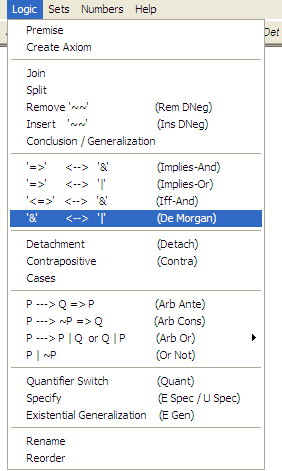### Features

• DC Proof is a free, downloadable, PC-based educational software program that can be used to learn basic proof-writing skills

• Recommended for use by...
• undergraduates in transition to proof-based mathematics
• teachers in training

• Simplified rules and axioms of logic and set theory
• based on common practices and simplifying assumptions implicit in most mathematics textbooks at the undergraduate level

• Color-coded variables indicate at a glance what generalizations can be made on them

• Convenient, pull-down menus enables you to apply any of the rules and axioms of logic, set theory and number theory with the click of a mouseFig. 1. Menu of the rules of Logic

• User comments and meaningful variable names (of unlimited length) facilitate both the reading and writing of proofs

Suppose that it is sunny if any only if it is it not cloudy.

1     Sunny <=> ~Cloudy

Premise

Fig. 2 User comment in blue. Line number and logical statement in black. Rule used in gray.

• Multi-level views of proofs that hide or reveal details as required ─ indispensible for writing longer proofs!

Top

#### Symbolic Logic and The Basic Methods of Proof

• DC Proof includes an interactive tutorial that introduces symbolic logic and the basic methods of proof using worked examples and exercises with hints and full solutions

• Topics in symbolic logic include:
• Logical propositions and predicates
• Logical operators
• Quantifiers ─ free and bound variables

• Methods of proof include:
• Direct proof
• Indirect proof
• Proof by contrapositive
• Proof by cases
• Proof of biconditionals
• Proof by induction
• Use of the formal axioms and definitions
• Set theory
• Elementary number theory

Examples of the Methods of Proof

 Tutorial Example DirectProof Proof byContradiction Proof byContrapositive Proof byCases Proof of Biconditionals ManipulatingQuantifiers Proof by Induction Set Theory Number Theory 1. The Commutativity of AND x 2. The Transitivity of IMPLIES x 3. The Commutativity of OR (by contradiction) x 4. The Commutativity of OR (by contrapositive) x 5. Proving a Biconditional x x x 6. The Distributivity of AND over OR  (Part 1) x x 7. The Transitivity of Equality x x 8. The Composition of Functions x x 9. The Barber Paradox x x x 10. The Paradox of the Universal Set x x x 11. The Uniqueness of the Empty Set x x x x x x 12. 1+1=2, 2+2=4 x x x x 13. No Number its own Successor x x x x x

Fig. 3 Table of methods of proof used in tutorial

Top